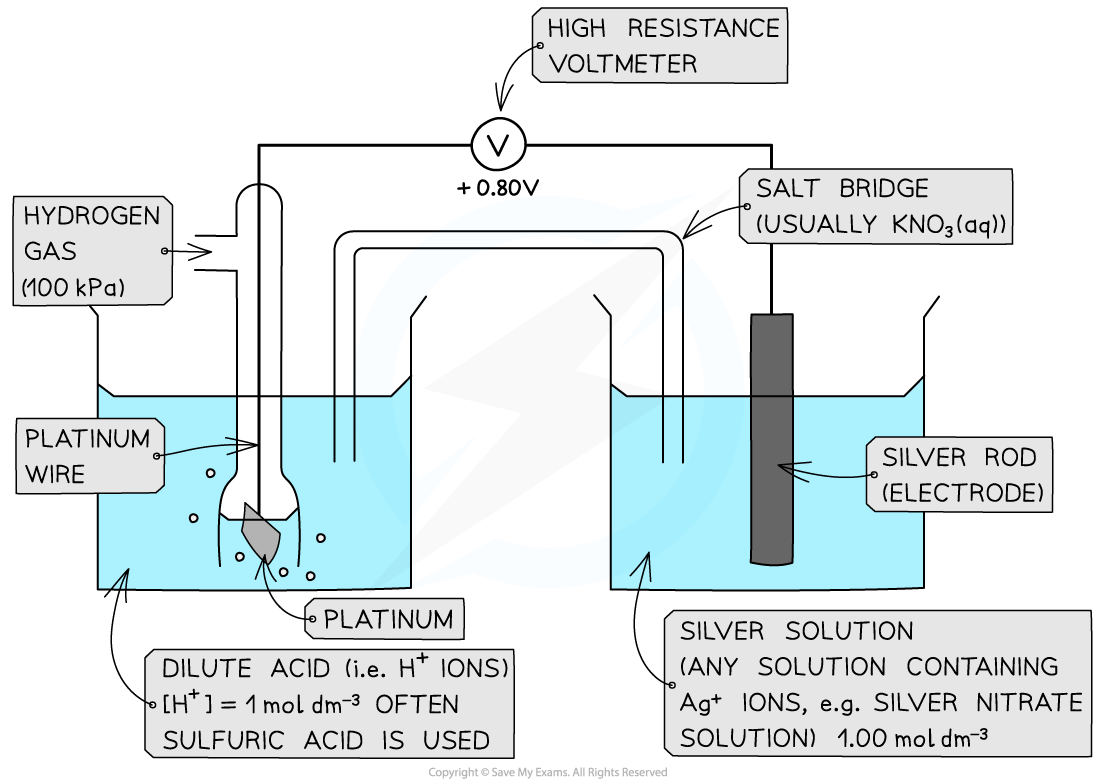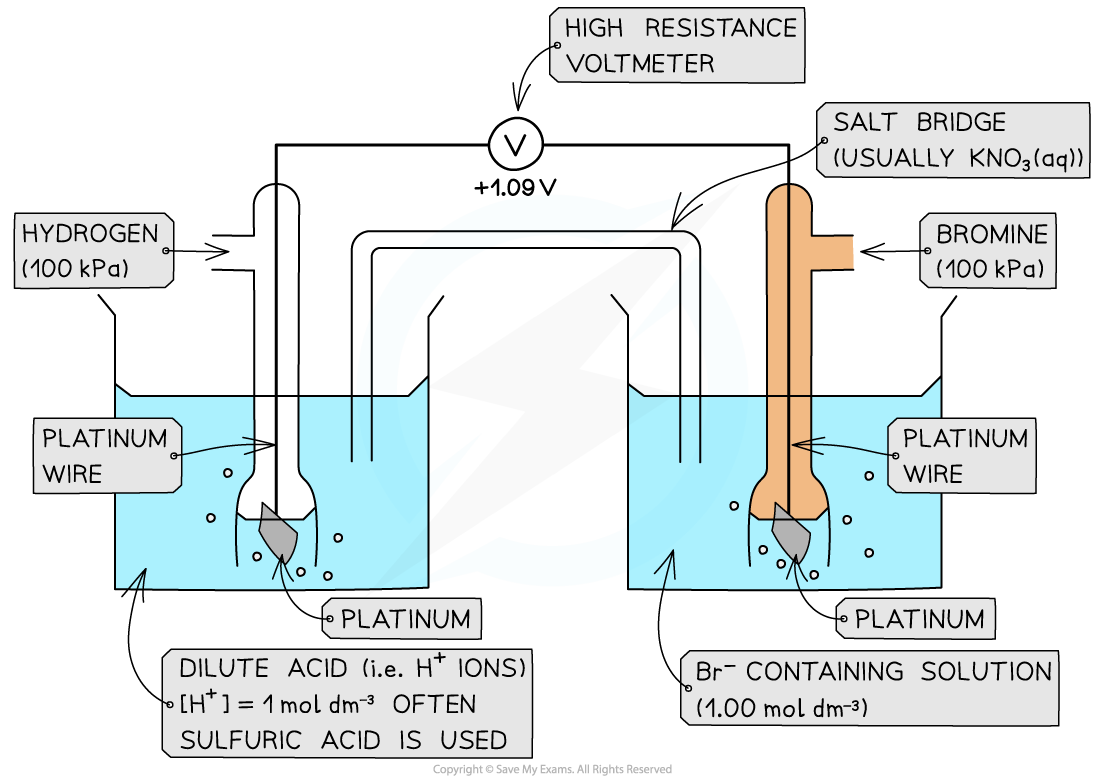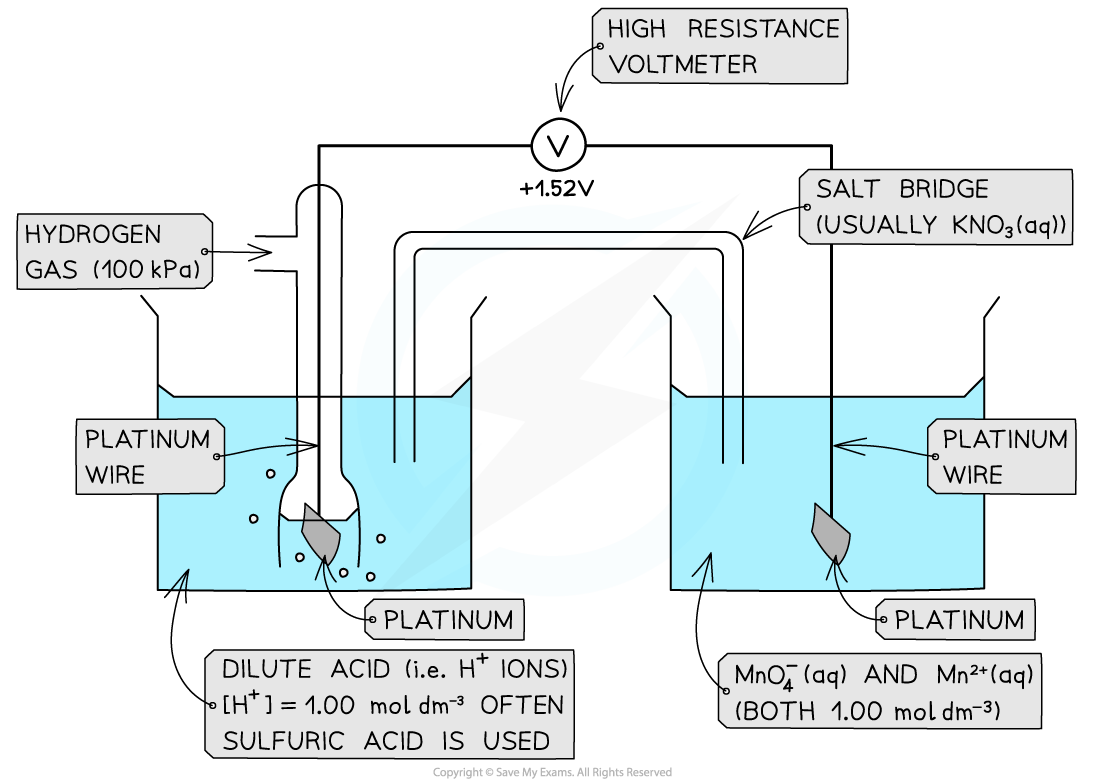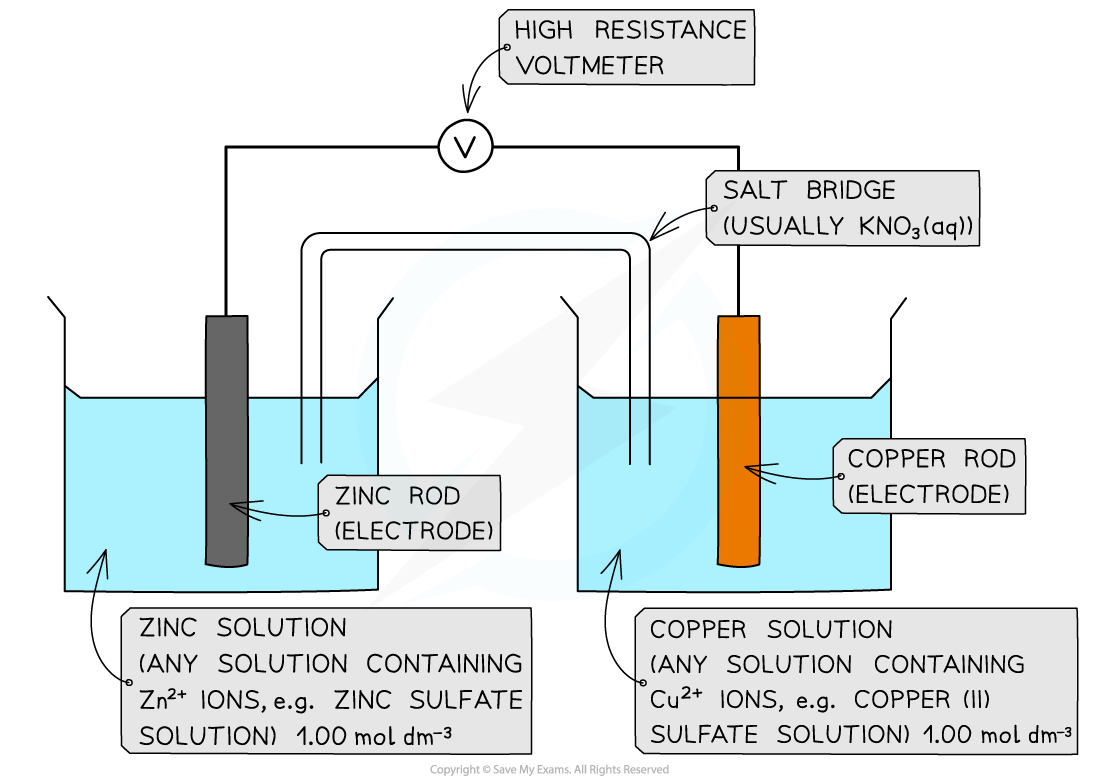# Edexcel A Level Chemistry:复习笔记6.1.3 Measuring Standard Electrode Potential

### Measuring Standard Electrode Potential

• There are three different types of half-cells that can be connected to a standard hydrogen electrode to measure standard electrode potential
• A metal / metal ion half-cell
• A non-metal / non-metal ion half-cell
• An ion / ion half-cell (the ions are in different oxidation states)

#### Metal / metal-ion half-cellExample of a metal / metal ion half-cell connected to a standard hydrogen electrode

• An example of a metal/metal ion half-cell is the Ag+/ Ag half-cell
• Ag is the metal
• Ag+ is the metal ion

• This half-cell is connected to a standard hydrogen electrode and the two half-equations are:

Ag+ (aq) + e- ⇌ Ag (s)        Eꝋ = + 0.80 V

2H+ (aq) + 2e- ⇌ H2 (g)        Eꝋ = 0.00 V

• Since the Ag+/ Ag half-cell has a more positive Eꝋ value, this is the positive pole and the H+/H2 half-cell is the negative pole
• The standard cell potential (Ecellꝋ) is Ecellꝋ = (+ 0.80) - (0.00) = + 0.80 V
• The Ag+ ions are more likely to get reduced than the H+ ions as it has a greater Eꝋ value
• Reduction occurs at the positive electrode
• Oxidation occurs at the negative electrode

#### Non-metal / non-metal ion half-cell

• In a non-metal / non-metal ion half-cell, platinum wire or foil is used as an electrode to make electrical contact with the solution
• Like graphite, platinum is inert and does not take part in the reaction
• The redox equilibrium is established on the platinum surface

• An example of a non-metal / non-metal ion is the Br2 / Br- half-cell
• Br2 is the non-metal
• Br- is the non-metal ion

• The half-cell is connected to a standard hydrogen electrode and the two half-equations are:

Br2 (aq) + 2e- ⇌ 2Br- (aq)        Eꝋ = +1.09 V

2H+ (aq) + 2e- ⇌ H2 (g)        Eꝋ = 0.00 V

• The Br2 / Br- half-cell is the positive pole and the H+ / H2 is the negative pole
• The Ecellꝋ is: Ecellꝋ = (+ 1.09) - (0.00) = + 1.09 V
• The Br2 molecules are more likely to get reduced than H+ as they have a greater Eꝋ valueExample of a non-metal / non-metal ion half-cell connected to a standard hydrogen electrode

#### Ion / Ion half-cell

• A platinum electrode is again used to form a half-cell of ions that are in different oxidation states
• An example of such a half-cell is the MnO4- / Mn2+ half-cell
• MnO4- is an ion containing Mn with oxidation state +7
• The Mn2+ ion contains Mn with oxidation state +2

• This half-cell is connected to a standard hydrogen electrode and the two half-equations are:

MnO4- (aq) + 8H+ (aq) + 5e- ⇌ Mn2+ (aq) + 4H2O (l)       Eꝋ = +1.52 V

2H+ (aq) + 2e- ⇌ H2 (g)       Eꝋ = 0.00 V

• The H+ ions are also present in the half-cell as they are required to convert MnO4- into Mn2+ ions
• The MnO4- / Mn2+ half-cell is the positive pole and the H+ / H2 is the negative pole
• The Ecellꝋ is Ecellꝋ = (+ 1.09) - (0.00) = + 1.09 VIons in solution half cell

### Electromotive Force

#### Standard cell potential

• Once the Eꝋ of a half-cell is known, the potential difference or voltage or emf of an electrochemical cell made up of any two half-cells can be calculated
• These could be any half-cells and neither have to be a standard hydrogen electrode

• The standard cell potential (Ecellꝋ) can be calculated by subtracting the less positive Eꝋ from the more positive Eꝋ value
• The half-cell with the more positive Eꝋ value will be the positive pole
• By convention this is shown on the right hand side in a conventional cell diagram, so is termed  Erightꝋ

• The half-cell with the less positive Eꝋ value will be the negative pole
• By convention this is shown on the left hand side in a conventional cell diagram, so is termed  Eleftꝋ

Ecellꝋ = Erightꝋ - Eleftꝋ

• Since oxidation is always on the left and reduction on the right, you can also use this version

Ecellꝋ = Ereductionꝋ - Eoxidation

#### Worked Example

Calculating the standard cell potential

Calculate the standard cell potential for the electrochemical cell below and explain why the Cu2+ / Cu half-cell is the positive pole. The half-equations are as follows:

Cu2+(aq) + 2e- ⇌ Cu(s)      Eꝋ = +0.34 V

Zn2+(aq) + 2e- ⇌ Zn(s)      Eꝋ = −0.76 VStep 1: Calculate the standard cell potential. The copper is more positive so must be the right hand side.

Ecellꝋ = Erightꝋ - Eleftꝋ

Ecellꝋ = (+0.34) - (-0.76)

= +1.10 V

The voltmeter will therefore give a value of +1.10 V

Step 2: Determine the positive and negative poles

The Cu2+ / Cu  half-cell is the positive pole as its Eꝋ is more positive than the Eꝋ value of the Zn2+ / Zn half-cell

#### Exam Tip

A helpful mnemonic for remembering redox in cellsLio the lion goes Roor!

Lio stands for 'Left Is Oxidation' and he is saying ROOR because that is the order of species in the cell:

Reduced/Oxidised (salt bridge) Oxidised/Reduced